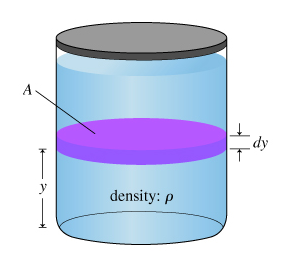# Problem: A. What is Fup, the magnitude of the force exerted upward on the bottom of the liquid?B. What is Fdown, the magnitude of the force exerted downward on the top of the liquid?C. What is the weight wlayer of the thin layer of liquid?Express your answer in terms of quantities given in the problem introduction and g, the magnitude of the acceleration due to gravity.D. Since the liquid is in equilibrium, the net force on the thin layer of liquid is zero. Complete the force equation for the sum of the vertical forces acting on the liquid layer described in the problem introduction.Express your answer in terms of quantities given in the problem introduction and taking upward forces to be positive.

###### FREE Expert Solution

A.

The pressure is expressed as:

$\overline{){\mathbf{P}}{\mathbf{=}}\frac{\mathbf{F}}{\mathbf{A}}}$, where F is the force acting on the liquid from the bottom, and A is the crossectional area of the container.

The force exerted upward on the bottom of the fluid is the force due to the pressure at the bottom of the liquid.

79% (289 ratings)###### Problem DetailsA. What is Fup, the magnitude of the force exerted upward on the bottom of the liquid?

B. What is Fdown, the magnitude of the force exerted downward on the top of the liquid?

C. What is the weight wlayer of the thin layer of liquid?

Express your answer in terms of quantities given in the problem introduction and g, the magnitude of the acceleration due to gravity.

D. Since the liquid is in equilibrium, the net force on the thin layer of liquid is zero. Complete the force equation for the sum of the vertical forces acting on the liquid layer described in the problem introduction.

Express your answer in terms of quantities given in the problem introduction and taking upward forces to be positive.

Frequently Asked Questions

What scientific concept do you need to know in order to solve this problem?

Our tutors have indicated that to solve this problem you will need to apply the Intro to Pressure concept. You can view video lessons to learn Intro to Pressure. Or if you need more Intro to Pressure practice, you can also practice Intro to Pressure practice problems.